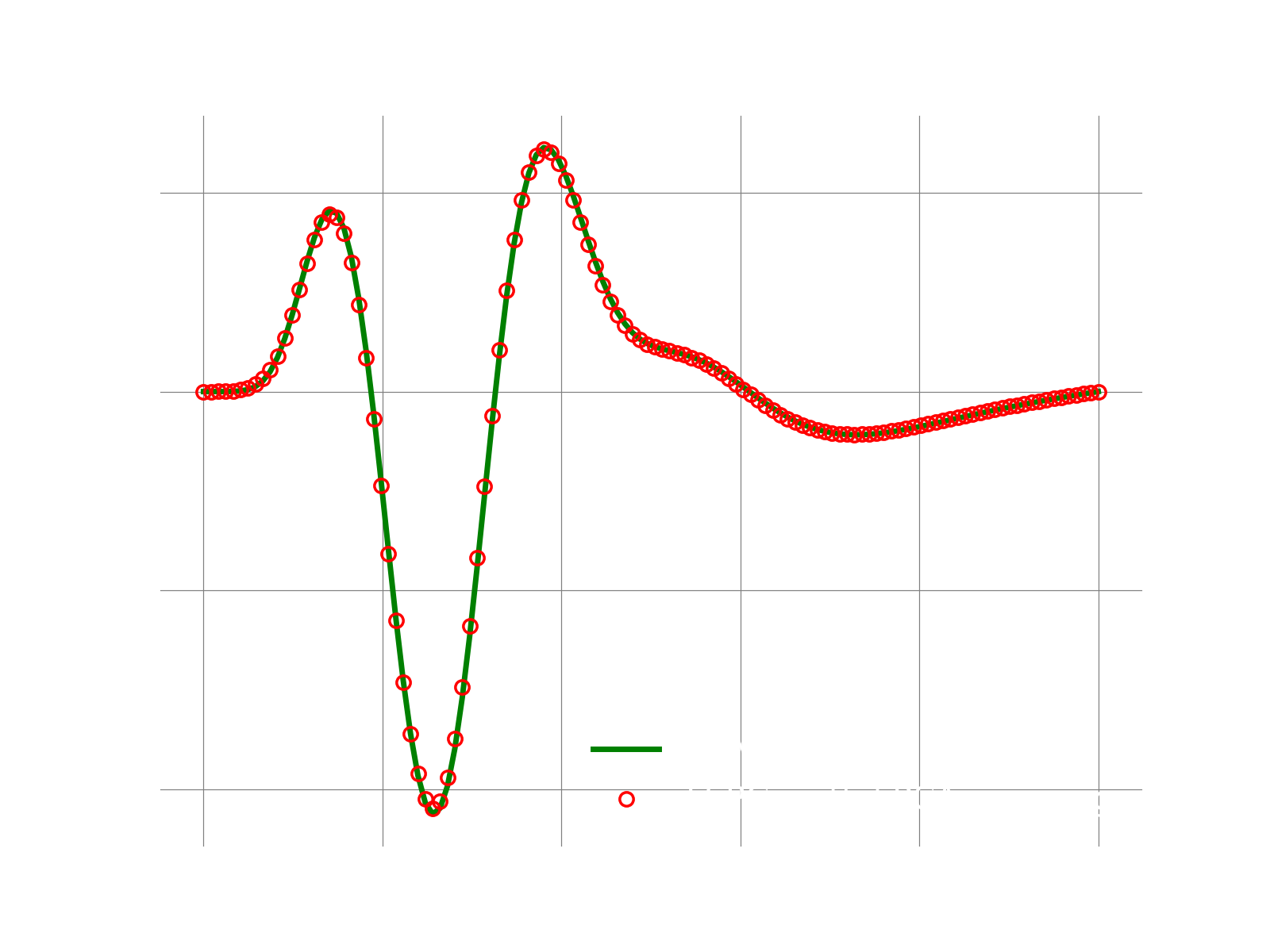# 1D adv-diff: LSPG with nonlinear manifold projection via kPCA

## Overview

This demo solves the same problem as the one here, but instead of using POD modes, we show here how to use a nonlinear manifold computed via kernel PCA.

## Main function

The main function of the demo is the following:

```logger.initialize(logger.logto.terminal)
logger.setVerbosity([logger.loglevel.info])

# create fom object

# the final time to integrate to
finalTime = .05

#--- 1. FOM ---#
fomTimeStepSize  = 1e-5
fomNumberOfSteps = int(finalTime/fomTimeStepSize)
sampleEvery      = 200
[fomFinalState, snapshots] = doFom(fomObj, fomTimeStepSize, fomNumberOfSteps, sampleEvery)

#--- 2. train a nonlinear mapping using kPCA ---#
# here we use 3 modes, change this to try different modes
myNonLinearMapper = MyMapperKPCA(snapshots.T, numModes=3)

#--- 3. LSPG ROM ---#
romTimeStepSize  = 3e-4
romNumberOfSteps = int(finalTime/romTimeStepSize)
approximatedState = runLspg(fomObj, romTimeStepSize, romNumberOfSteps, myNonLinearMapper)

# compute l2-error between fom and approximate state
fomNorm = linalg.norm(fomFinalState)
err = linalg.norm(fomFinalState-approximatedState)
print("Final state relative l2 error: {}".format(err/fomNorm))

logger.finalize()```

### 1. Run FOM and collect snapshots

This step is the same as described here,

### 2. Setup and train the nonlinear kPCA mapper

It is important to note that while the mapper class below has the API required by pressio4py, it can encapsulate any arbitrary mapping function. In this case we show how to create a kPCA-based representation, but one can use, e.g., autoencoder, and any other types of mapping. This is how we enable support for testing various methods.

```class MyMapperKPCA:
def __init__(self, snapshots, numModes):
self.transformer_ = skd.KernelPCA(n_components=numModes,\
kernel='poly',
degree=3,
fit_inverse_transform=True)
# do training using provided snapshots
self.transformer_.fit(snapshots)

self.numModes_ = numModes
fomSize = snapshots.shape
self.fomState0 = np.zeros(fomSize)
self.fomState1 = np.zeros(fomSize)
# attention: the jacobian of the mapping must be column-major oder
# so that pressio can view it without deep copying it, this enables
# to keep only one jacobian object around and to call the update
# method below correctly
self.jacobian_ = np.zeros((fomSize,numModes), order='F')

def numModes(self): return self.numModes_

def jacobian(self): return self.jacobian_

def applyMapping(self, romState, fomState):
fomState[:] = np.squeeze(self.transformer_.inverse_transform(romState.reshape(1,-1)))

def applyInverseMapping(self, romState, fomState):
romState[:] = np.squeeze(self.transformer_.transform(fomState.reshape(1,-1)))

def updateJacobian(self, romState):
romStateLocal = romState.copy()
# finite difference to approximate jacobian of the mapping
self.applyMapping(romStateLocal,self.fomState0)
eps = 0.001
for i in range(self.numModes_):
romStateLocal[i] += eps
self.applyMapping(romStateLocal, self.fomState1)
self.jacobian_[:,i] = (self.fomState1 - self.fomState0) / eps
romStateLocal[i] -= eps```

### 3. Construct and run LSPG

```def runLspg(fomObj, dt, nsteps, customMapper):
# this is an auxiliary class that can be passed to solve
# LSPG to monitor the rom state.
class RomStateObserver:
def __call__(self, timeStep, time, state): pass

# this linear solver is used at each gauss-newton iteration
class MyLinSolver:
def solve(self, A,b,x):
lumat, piv, info = linalg.lapack.dgetrf(A, overwrite_a=True)
x[:], info = linalg.lapack.dgetrs(lumat, piv, b, 0, 0)

#----------------------------------------
# create a custom decoder using the mapper passed as argument
customDecoder = rom.Decoder(customMapper, "kPCAMapper")

# fom reference state: here it is zero
fomReferenceState = np.zeros(fomObj.nGrid)

# create ROM state by projecting the fom initial condition
fomInitialState = fomObj.u0.copy()
romState = np.zeros(customMapper.numModes())
customMapper.applyInverseMapping(romState, fomInitialState)

# create LSPG problem
scheme = ode.stepscheme.BDF1
problem = rom.lspg.unsteady.DefaultProblem(scheme, fomObj, customDecoder, romState, fomReferenceState)

# create the Gauss-Newton solver
nonLinSolver = solvers.create_gauss_newton(problem, romState, MyLinSolver())
# set tolerance and convergence criteria
nlsTol, nlsMaxIt = 1e-7, 10
nonLinSolver.setMaxIterations(nlsMaxIt)
nonLinSolver.setStoppingCriterion(solvers.stop.WhenCorrectionAbsoluteNormBelowTolerance)

# create object to monitor the romState at every iteration
myObs = RomStateObserver()
# solve problem
ode.advance_n_steps_and_observe(problem, romState, 0., dt, nsteps, myObs, nonLinSolver)

# after we are done, use the reconstructor object to reconstruct the fom state
# get the reconstructor object: this allows to map romState to fomState
fomRecon = problem.fomStateReconstructor()
return fomRecon(romState)```

## Results

If everything works fine, the following plot shows the result.